# Pandas AI: The Generative AI Python Library

In the age of AI, many of our tasks have been automated especially after the launch of ChatGPT. One such tool that uses the power of ChatGPT to ease data manipulation task in Python is PandasAI. It leverages the power of ChatGPT to generate Python code and executes it. The output of the generated code is returned. Pandas AI helps performing tasks involving pandas library without explicitly writing lines of code. In this article we will discuss about how one can use Pandas AI to simplify data manipulation.

## What is Pandas AI

Using generative AI models from OpenAI, Pandas AI is a pandas library addition. With simply a text prompt, you can produce insights from your dataframe. It utilises the OpenAI-developed text-to-query generative AI. The preparation of the data for analysis is a labor-intensive process for data scientists and analysts. Now they can carry on with their data analysis. Data experts may now leverage many of the methods and techniques they have studied to cut down on the time needed for data preparation thanks to Pandas AI. PandasAI should be used in conjunction with Pandas, not as a substitute for Pandas. Instead of having to manually traverse the dataset and react to inquiries about it, you can ask PandasAI these questions, and it will provide you answers in the form of Pandas DataFrames. Pandas AI wants to make it possible for you to visually communicate with a machine that will then deliver the desired results rather than having to program the work yourself. To do this, it uses the OpenAI GPT API to generate the code using Pandas library in Python and run this code in the background. The results are then returned which can be saved inside a variable.

### How Can I use Pandas AI in my projects

#### 1. Install and Import of Pandas AI library in python environment

Execute the following command in your jupyter notebook to install pandasai library in python

`!pip install -q pandasai`

Import pandasai library in python

## Python3

 `import` `pandas as pd ` `import` `numpy as np ` `from` `pandasai ``import` `PandasAI ` `from` `pandasai.llm.openai ``import` `OpenAI`

### 2. Add data to an empty DataFrame

Make a dataframe using a dictionary with dummy data

## Python3

 `data_dict ``=` `{ ` `    ``"country"``: [ ` `        ``"Delhi"``, ` `        ``"Mumbai"``, ` `        ``"Kolkata"``, ` `        ``"Chennai"``, ` `        ``"Jaipur"``, ` `        ``"Lucknow"``, ` `        ``"Pune"``, ` `        ``"Bengaluru"``, ` `        ``"Amritsar"``, ` `        ``"Agra"``, ` `        ``"Kola"``, ` `    ``], ` `    ``"annual tax collected"``: [ ` `        ``19294482072``, ` `        ``28916155672``, ` `        ``24112550372``, ` `        ``34358173362``, ` `        ``17454337886``, ` `        ``11812051350``, ` `        ``16074023894``, ` `        ``14909678554``, ` `        ``43807565410``, ` `        ``146318441864``, ` `        ``np.nan, ` `    ``], ` `    ``"happiness_index"``: [``9.94``, ``7.16``, ``6.35``, ``8.07``, ``6.98``, ``6.1``, ``4.23``, ``8.22``, ``6.87``, ``3.36``, np.nan], ` `} ` ` `  `df ``=` `pd.DataFrame(data_dict) ` `df.head()`

Output: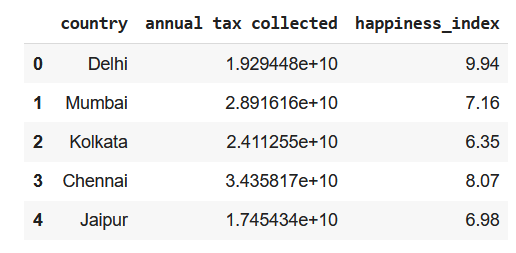First 5 rows of the DataFrame

## Python3

 `df.tail()`

Output: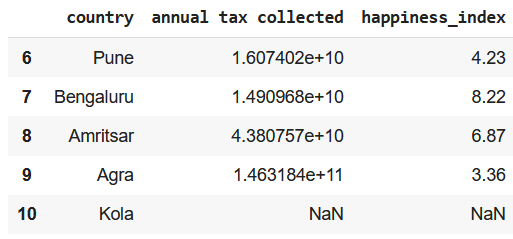Last 5 rows of DataFrame

## Python3

 `llm ``=` `OpenAI(api_token``=``"API_KEY"``) ` `pandas_ai ``=` `PandasAI(llm, conversational``=``False``)`

#### 4. Trying pandas features using pandasai

Prompt 1: Finding index of a value

## Python3

 `# finding index of a row using value of a column ` `response ``=` `pandas_ai(df, ``"What is the index of Pune?"``) ` `print``(response)`

Output:

`6`

Prompt 2: Using Head() function of DataFrame

## Python3

 `response ``=` `pandas_ai(df, ``"Show the first 5 rows of data in tabular form"``) ` `print``(response)`

Output:

`    country  annual tax collected  happiness_index0    Delhi          1.929448e+10             9.941   Mumbai          2.891616e+10             7.162  Kolkata          2.411255e+10             6.353  Chennai          3.435817e+10             8.074   Jaipur          1.745434e+10             6.98`

Prompt 3: Using Tail() function of DataFrame

## Python3

 `response ``=` `pandas_ai(df, ``"Show the last 5 rows of data in tabular form"``) ` `print``(response)`

Output:

`      country  annual tax collected  happiness_index6        Pune          1.607402e+10             4.237   Bengaluru          1.490968e+10             8.228    Amritsar          4.380757e+10             6.879        Agra          1.463184e+11             3.3610       Kola                   NaN              NaN`

Prompt 4: Using describe() function of DataFrame

## Python3

 `response ``=` `pandas_ai(df, ``"Show the description of data in tabular form"``) ` `print``(response)`

Output:

`        annual tax collected  happiness_indexcount          1.000000e+01        10.000000mean           3.570575e+10         6.728000std            4.010314e+10         1.907149min            1.181205e+10         3.36000025%            1.641910e+10         6.16250050%            2.170352e+10         6.92500075%            3.299767e+10         7.842500max            1.463184e+11         9.940000`

Prompt 5: Using the info() function of DataFrame

## Python3

 `response ``=` `pandas_ai(df, ``"Show the info of data in tabular form"``) ` `print``(response)`

Output:

`<class 'pandas.core.frame.DataFrame'>Index: 11 entries, 0 to 10Data columns (total 3 columns): #   Column                Non-Null Count  Dtype  ---  ------                --------------  -----   0   Country               11 non-null     object  1   annual tax collected  11 non-null     float64 2   happiness_index       11 non-null     float64dtypes: float64(2), object(1)memory usage: 652.0+ bytes`

Prompt 6: Using shape attribute of dataframe

## Python3

 `response ``=` `pandas_ai(df, ``"What is the shape of data?"``) ` `print``(response)`

Output:

`(11, 3)`

Prompt 7: Finding any duplicate rows

## Python3

 `response ``=` `pandas_ai(df, ``"Are there any duplicate rows?"``) ` `print``(response)`

Output:

`There are no duplicate rows.`

Prompt 8: Finding missing values

## Python3

 `response ``=` `pandas_ai(df, ``"Are there any missing values?"``) ` `print``(response)`

Output:

`False`

Prompt 9: Drop rows with missing values

## Python3

 `response ``=` `pandas_ai(df, ``"Drop the row with missing values with inplace=True and return True when done else False "``) ` `print``(response)`

Output:

`False`

Checking if the last has been removed row

## Python3

 `df.tail()`

Output: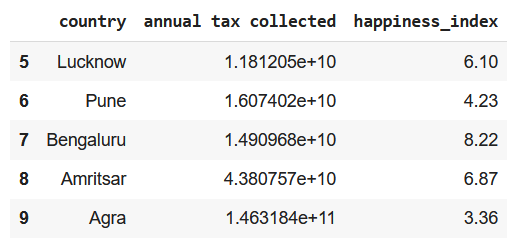Last row has been removed because it had Nan values

Prompt 10: Print all column names

## Python3

 `response ``=` `pandas_ai(df, ``"List all the column names"``) ` `print``(response)`

Output:

`['country', 'annual tax collected', 'happiness_index']`

Prompt 11: Rename a column

## Python3

 `response ``=` `pandas_ai(df, ``"Rename column 'country' as 'Country' keep inplace=True and list all column names"``) ` `print``(response)`

Output:

`Index(['Country', 'annual tax collected', 'happiness_index'], dtype='object')`

Prompt 12: Add a row at the end of the dataframe

## Python3

 `response ``=` `pandas_ai(df, ``"Add the list: ['A',None,None] at the end of the dataframe as last row keep inplace=True"``) ` `print``(response)`

Output:

`      Country  annual tax collected  happiness_index0       Delhi          1.929448e+10             9.941      Mumbai          2.891616e+10             7.162     Kolkata          2.411255e+10             6.353     Chennai          3.435817e+10             8.074      Jaipur          1.745434e+10             6.985     Lucknow          1.181205e+10             6.106        Pune          1.607402e+10             4.237   Bengaluru          1.490968e+10             8.228    Amritsar          4.380757e+10             6.879        Agra          1.463184e+11             3.3610          A                   NaN              NaN`

Prompt 13: Replace the missing values

## Python3

 `response ``=` `pandas_ai(df, ``"""Fill the NULL values in dataframe with 0 keep inplace=True  ` `and the print the last row of dataframe"""``) ` `print``(response)`

Output:

`   Country  annual tax collected  happiness_index10       A                   0.0              0.0`

Prompt 14: Calculating mean of a column

## Python3

 `response ``=` `pandas_ai(df, ``"What is the mean of annual tax collected"``) ` `print``(response)`

Output:

`32459769130.545456`

Prompt 15: Finding frequency of unique values of a column

## Python3

 `response ``=` `pandas_ai(df, ``"What are the value counts for the column 'Country'"``) ` `print``(response)`

Output:

`CountryDelhi        1Mumbai       1Kolkata      1Chennai      1Jaipur       1Lucknow      1Pune         1Bengaluru    1Amritsar     1Agra         1A            1Name: count, dtype: int64`

Prompt 16: Dataframe Slicing

## Python3

 `response ``=` `pandas_ai(df, ``"Show first 3 rows of columns 'Country' and 'happiness index'"``) ` `print``(response)`

Output:

`   Country  happiness_index0    Delhi             9.941   Mumbai             7.162  Kolkata             6.35`

Prompt 17: Using pandas where function

## Python3

 `response ``=` `pandas_ai(df, ``"Show the data in the row where 'Country'='Mumbai'"``) ` `print``(response)`

Output:

`  Country  annual tax collected  happiness_index1  Mumbai          2.891616e+10             7.16`

Prompt 18: Using pandas where function with a range of values

## Python3

 `response ``=` `pandas_ai(df, ``"Show the rows where 'happiness index' is between 3 and 6"``) ` `print``(response)`

Output:

`  Country  annual tax collected  happiness_index 6    Pune          1.607402e+10             4.239    Agra          1.463184e+11             3.36`

Prompt 19: Finding 25th percentile of a column of continuous values

## Python3

 `response ``=` `pandas_ai(df, ``"What is the 25th percentile value of 'happiness index'"``) ` `print``(response)`

Output:

`5.165`

Prompt 20: Finding IQR of a column

## Python3

 `response ``=` `pandas_ai(df, ``"What is the IQR value of 'happiness index'"``) ` `print``(response)`

Output:

`2.45`

Prompt 21: Plotting a box plot for a continuous column

## Python3

 `response ``=` `pandas_ai(df, ``"Plot a box plot for the column 'happiness index'"``) ` `print``(response)`

Output: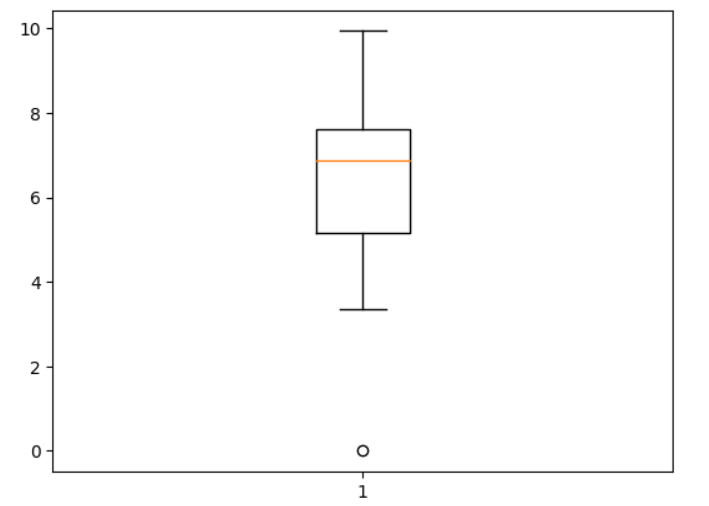Box plot of Happiness Index using PandasAI

Prompt 22: Find outliers in a column

## Python3

 `response ``=` `pandas_ai(df, ``"Show the data of the outlier value in the columns 'happiness index'"``) ` `print``(response)`

Output:

`  Country  annual tax collected  happiness_index0   Delhi          1.929448e+10             9.94`

Prompt 23: Plot a scatter plot between 2 columns

## Python3

 `response ``=` `pandas_ai(df, ``"Plot a scatter plot for the columns'annual tax collected' and 'happiness index'"``) ` `print``(response)`

Output: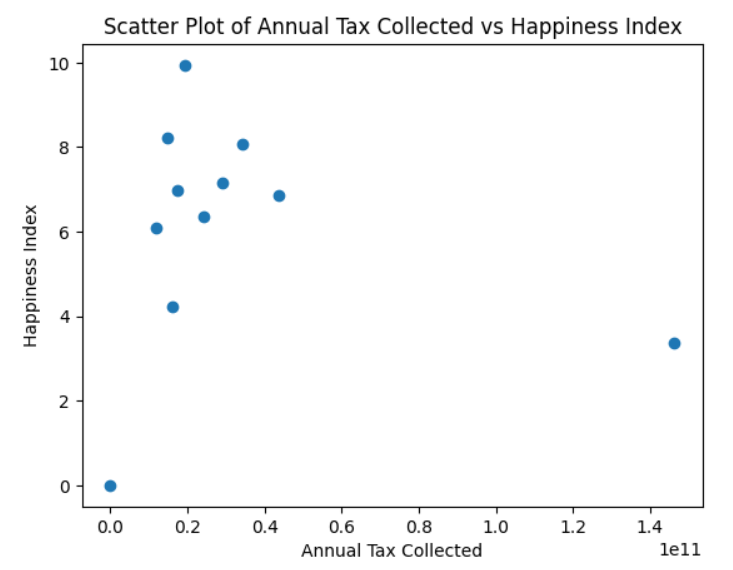Scatter plot of Happiness Index and Annual Tax Collected using Pandas AI

Prompt 24: Describing a column/series

## Python3

 `response ``=` `pandas_ai(df, ``"Describe the column 'annual tax collected'"``) ` `print``(response)`

Output:

`count    1.100000e+01mean     3.245977e+10std      3.953904e+10min      0.000000e+0025%      1.549185e+1050%      1.929448e+1075%      3.163716e+10max      1.463184e+11Name: annual tax collected, dtype: float64`

Prompt 25: Plot a bar plot between 2 columns

## Python3

 `response ``=` `pandas_ai(df, ``"Plot a bar plot for the columns'annual tax collected' and 'Country'"``) ` `print``(response)`

Output: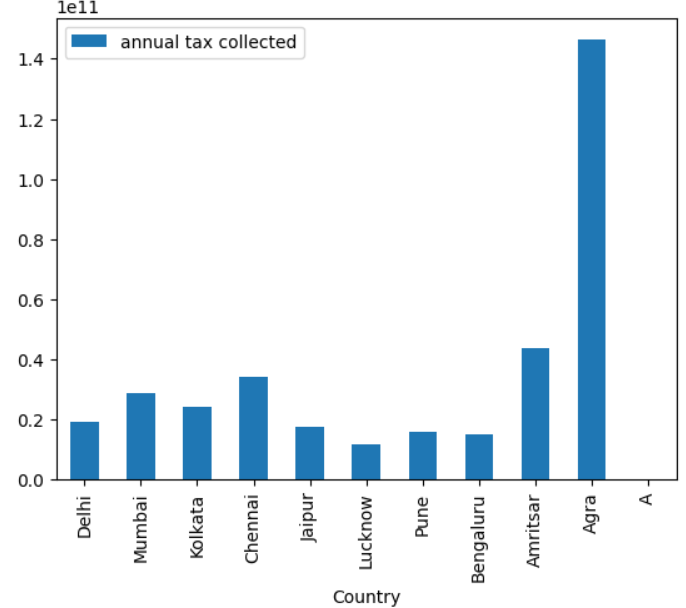Bar plot between Country and Tax Collected using Pandas AI

Prompt 26: Saving DataFrame as a CSV file and JSON file

## Python3

 `# to save the dataframe as a CSV file ` `response ``=` `pandas_ai(df, ``"Save the dataframe to 'temp.csv'"``) ` `# to save the dataframe as a JSON file ` `response ``=` `pandas_ai(df, ``"Save the dataframe to 'temp.json'"``)`

These lines of code will save your DataFrame as a CSV file and JSON file.

## Pros and Cons of Pandas AI

Pros of Pandas AI

• Can easily perform simple tasks without having to remember any complex syntax
• Capable of giving conversational replies
• Easy report generation for quick analysis or data manipulation

Cons of Pandas AI

• Cannot create or interact with variables other than the passed dataframe

1. Is Pandas AI replacing Pandas ?

No, Pandas AI is not meant to replace Pandas. Though Pandas AI can easily perform simple tasks, it still faces difficulty performing some complex tasks like saving the dataframe, making a correlation matrix and many more. Pandas AI is best for quick analysis, data cleaning and data manipulation but when we have to perform some complex functions like join, save dataframe, read a file, or create a correlation matrix we should prefer Pandas. Pandas AI is just an extension of Pandas, for now it cannot replace Pandas.

2. When to use Pandas AI ?

For simple tasks one could consider using Pandas AI, here you won’t have to remember any syntax. All you have to do is design a very descriptive prompt and rest will be done by Open AI’s LLM. But if you want to perform some complex tasks, you should prefer using Pandas.

3. How does Pandas AI work in the backend?

Pandas AI takes in the dataframe and your query as input and passes it to a collection of OpenAI’s LLM’s. Pandas AI uses ChatGPT’s API in the backend to generate the code and executes it. The output after execution is returned to you.

4. Can PandasAI work without OpenAI’s API?

Yes, other than ChatGPT you can also use Google’s PaLm model, Open Assistant LLM and StarCoder LLM for code generation.

5. Which to use Pandas or PandasAI for Exploratory Data Analysis?

You can first try using PandasAI to check if the data is good to perform an in depth analysis, then you can perform an in-depth analysis using Pandas and other libraries.

6. Can PandasAI use numpy attributes or functions?

No, it does not have the ability to use numpy functions. All computations are performed either by using Pandas or in-built python functions in the backend.

## Conclusion

In this article we focused on how to use PandasAI to perform all the major functionality supported by Pandas to perform a quick analysis on your dataset. By automating several operations, it without a doubt boosts productivity. It’s important to keep in mind that even though PandasAI is a powerful tool, the Pandas library must still be used. PandasAI is therefore a beneficial addition that improves the capability of the pandas library and further increases the effectiveness and simplicity of dealing with data in Python.

Whether you're preparing for your first job interview or aiming to upskill in this ever-evolving tech landscape, GeeksforGeeks Courses are your key to success. We provide top-quality content at affordable prices, all geared towards accelerating your growth in a time-bound manner. Join the millions we've already empowered, and we're here to do the same for you. Don't miss out - check it out now!